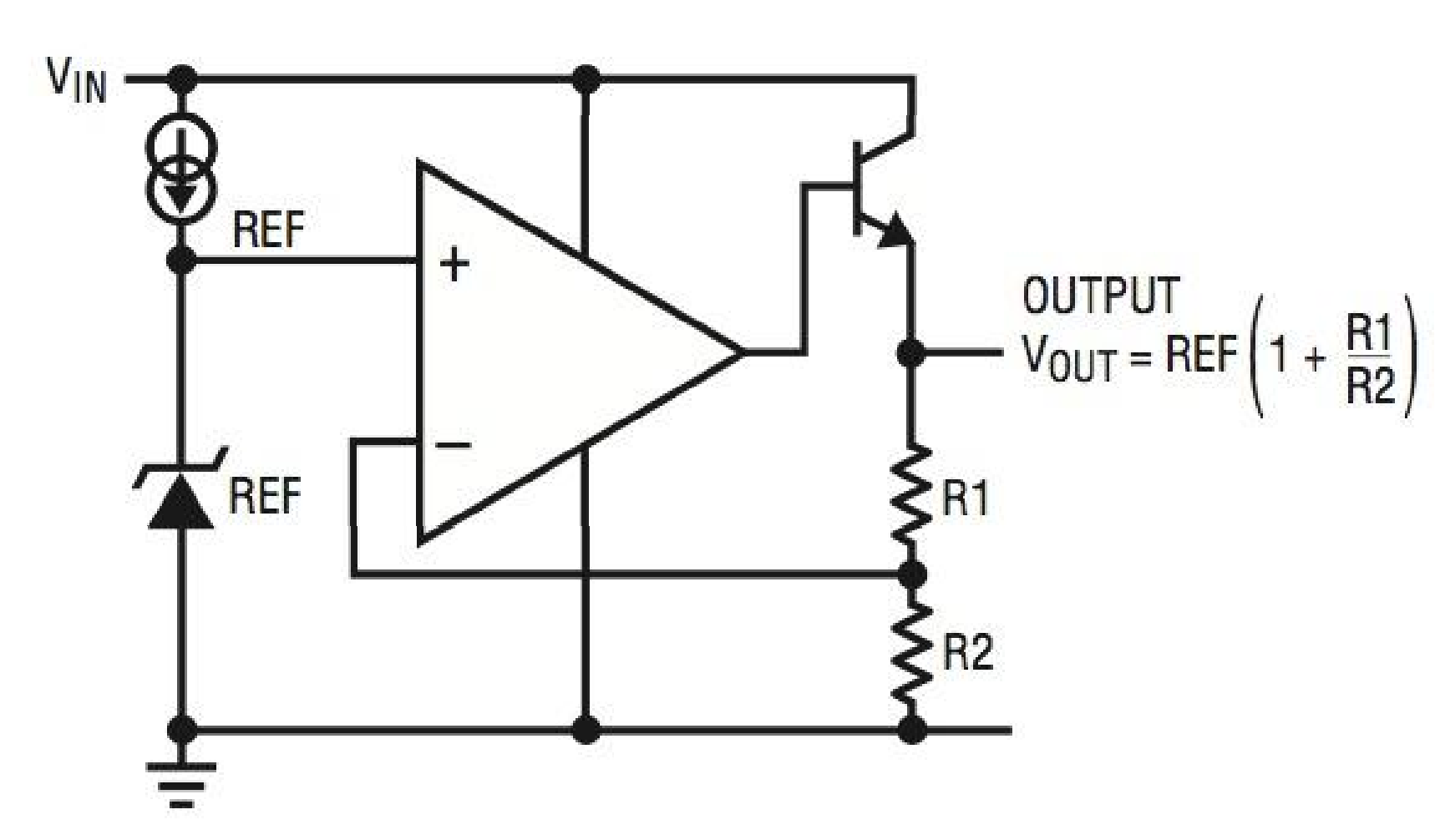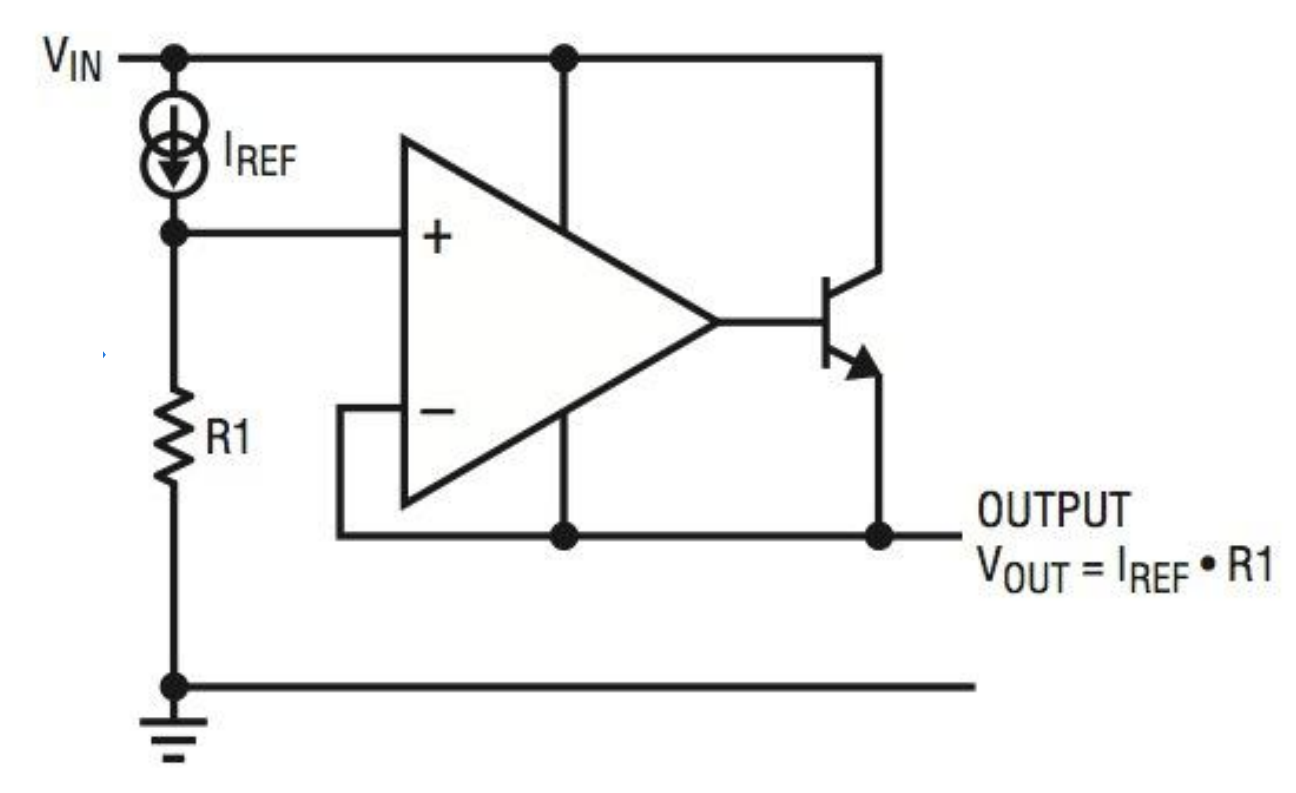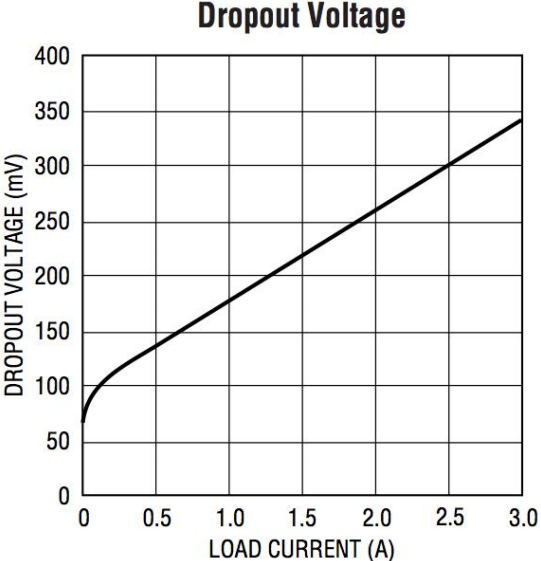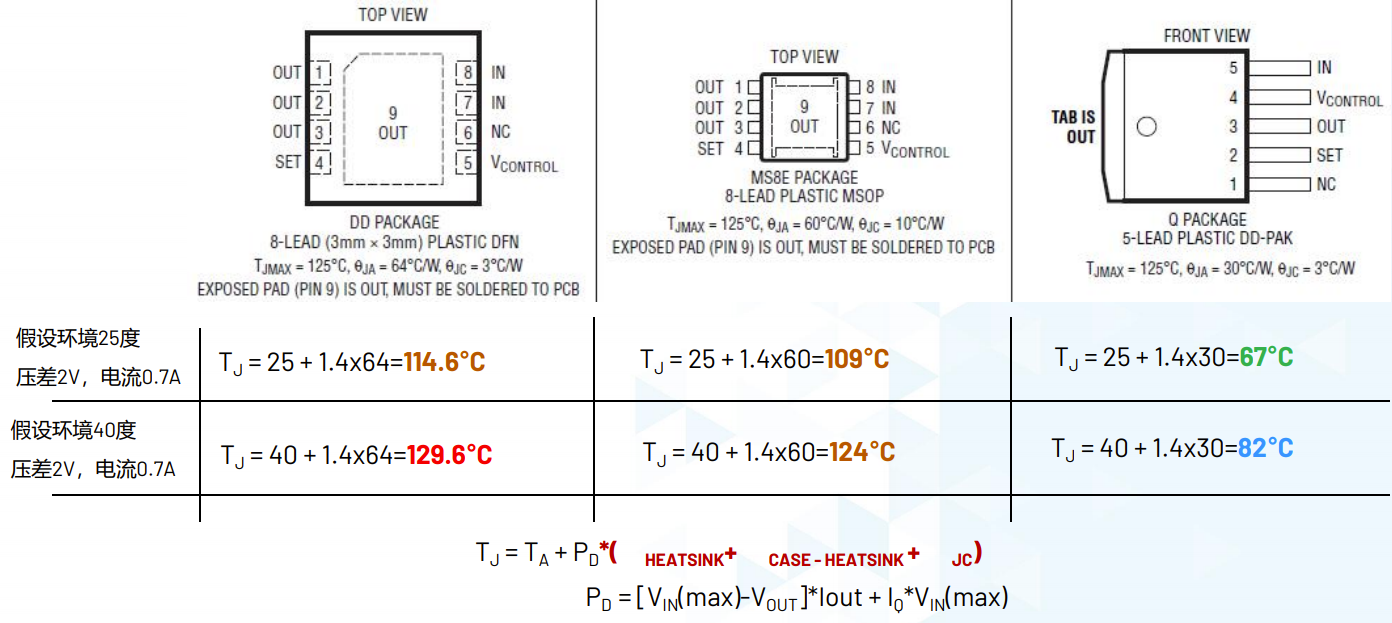# 线性稳压器LDO

## 线性稳压器(LDO)优缺点

1. 简单易用，外围器件少
2. 具有较低的噪声
3. 具有快速的瞬态响应

### LDO缺点

1. 线性稳压器不能够升压，输出电压必须低于输入电压，即$V_o
2. 当输入电压与输出电压压差较大时，LDO的效率较低，热耗较大，发热是个大问题。

## LDO的两种架构

### 传统LDO架构

• 在1976年，LTC创始人Robert Dobkin 和Bob Widlar设计了第一个推向市场的线性变换器LM317
• 通过稳压管在内部稳压出一个参考电压输出到内部集成运算放大器的负反馈端作为电压基准；将LDO的输出电压通过电阻R1和R2分压出一个参考电压接到放大器的正反馈端，再将放大器的输出端接在三极管的基极调节三极管的导通状况。
• 回路中的晶体管运行于线性区，这就像在输入级与输出级之间放置了一个可调电阻，勉强承受两个节点之间的电压降。### 新LDO架构

• 新一代架构的LDO在内部设计了一个精确的电流源基准，将通过电阻R1产生的电压接至内部集成运算放大器的负反馈端作为电压基准，将LDO的输出电压直接接至放大器的正反馈端，再将放大器的输出端接在三极管的基极调节三极管的导通状况。
1. 相比旧的架构，极大改善了电压调节能力和瞬态响应。
2. 将输出电压降低至零成为可能
3. 可以通过非常简单的并联获得更大的输出电流和用户自定义的电流限制。## LDO的效率问题

• 对于LDO芯片而言，输出电流近乎等于输入电流，输出电压与输入电压之间的压差全部损耗在内部三极管上转化为了热能。
• 所以当输入电压与输出电压压降较大时，LDO的效率则较低；而当输入电压与输出电压压降较为接近时，LDO则可以获得一个较高的效率。

$\eta _{LR} =\frac{P_O}{P_{IN}} =\frac{V_O}{V_{IN}}$

## LDO电压降

• 压降(VDD)：输入与输出之间能够维持正常工作的最小压差。
• 对于LDO稳压输出,要求输入电压必须比输出电压高，即$V_{IN}=V_{OUT}+V_{DO}$
• 对于普通的LDO器件，如LM7805芯片，至少需要2V以上的压差；对于低压差LDO器件，通常压差小于1V(300mV较为常见)；而对于极低压差LDO，则可以做到小于100mV的压差，如LT3701在5A输出时只有85mV的压差。
• 还需注意，压差的大小与能够输出的最大电流之间有直接关系。如下图所示的关系表，在输出1A电流时，只需要约175mV左右的压差；而当输出2.5A电流时，则需要300mA的压差。# LDO的输入输出电容

• 对于输入电容($C_{IN}$)，需要选择合适的大小输入电容，以防止LDO在瞬态突变负载时进入跌落状态。
• 对于输出电容($C_{OUT}$)，电容的大小与类型若没有合理选择，会影响LDO的输出稳定性与瞬态响应。
• 一般来说，较大输出电容值($C_{OUT}$)会减少峰值偏移，改善瞬态响应；且常用不同类型的电容器并联组合使用，以获得更好的瞬态响应。
• 具体的输入输出电容值大小及类型，一般在LDO器件的数据手册中都会有明确说明，在进行电路设计时需加以注意。

# 损耗功率与热问题

## 最大功率损耗($P_D$)

$P_D=[V_{IN}(max)-V_{OUT}] \times I_{OUT}+I_Q \times V_{IN}(max)$

$P_D$ 最大功率损耗
$V_{IN}(max)$ LDO的最大输入电压
$V_{OUT}$ LDO的输出电压
$I_{OUT}$ LDO的输出电流
$I_Q$ LDO的静态工作电压

## 结温

$T_J = T_A+P_D \times ( {\theta}_{HEATSINK} + {\theta}_{CASE-HEATSINK} + {\theta}_{JC})$

$T_J$ 结温
$T_A$ 环境温度
${\theta}_{HEATSINK}$ 散热器到空间热阻
${\theta}_{CASE-HEATSINK}$ 壳体到散热器热阻
${\theta}_{JC}$ 内核到外壳热阻
• 为芯片添加散热器的目的：确保内核温度$T_J$不会超过最大的规格书标定的可以正常运行的结温$T_J$
• 对于不同的封装形式，有着不同大小的热阻，我们应依照实际工况的最大功率损耗$P_D$，选择合适的封装形式。## 常用LDO封装功耗限制

• 以下功耗封装限制基于PCB最高环境温度为60℃，和最大硅晶体温度为125℃为前提条件而得到。

SOT-23-5 0.3W
SOT-89-3 0.5W
SOT-223 0.6W
PSOP-8 0.9W
TO-252-3 1.2W